# Linear algebra behind Google search

Teaching um VAST, Thrissur, India
18. Aug 2015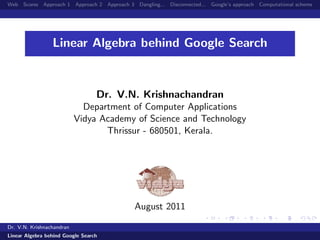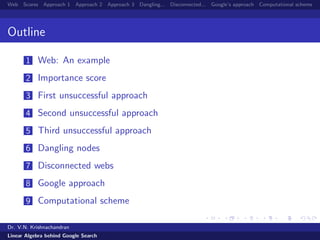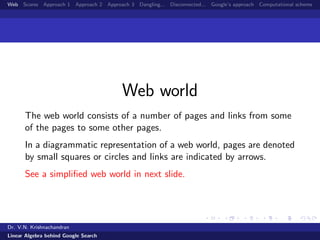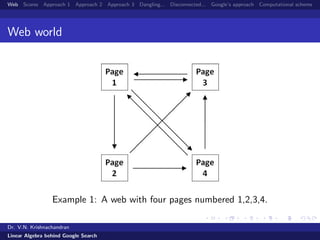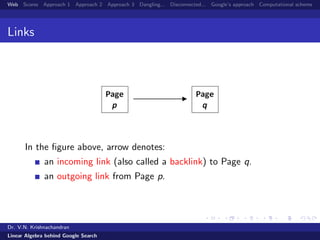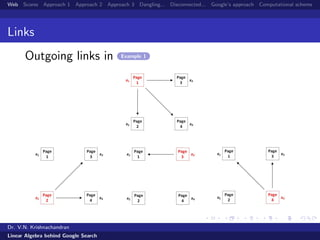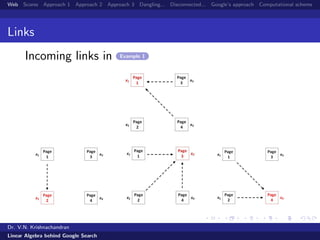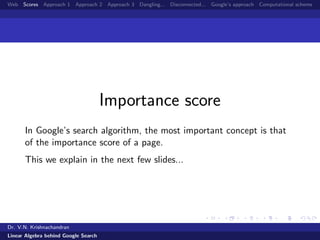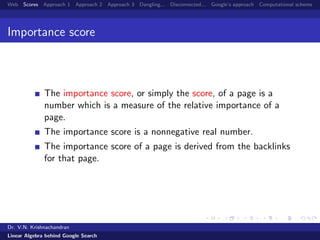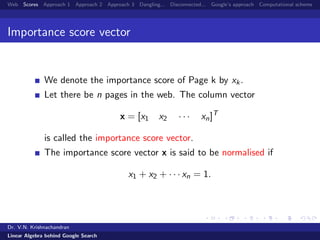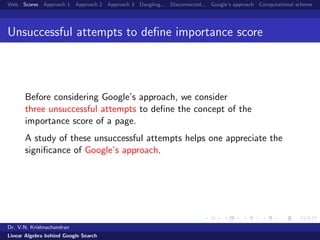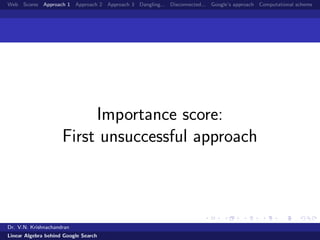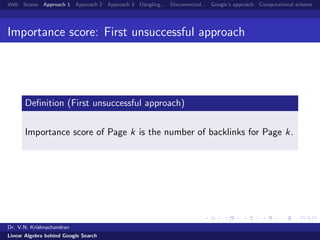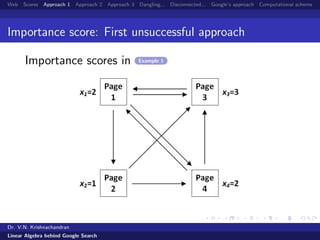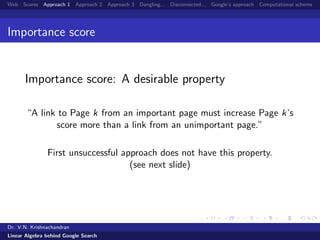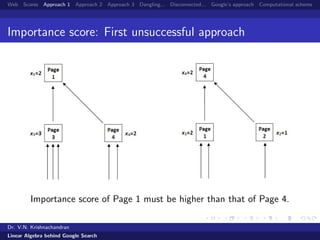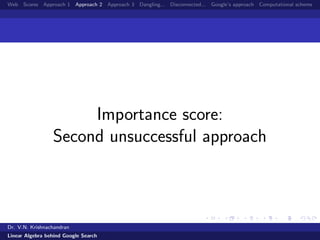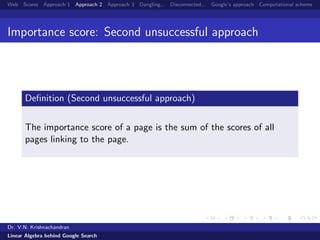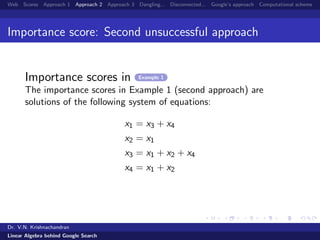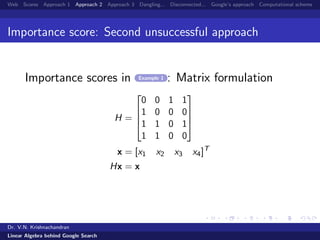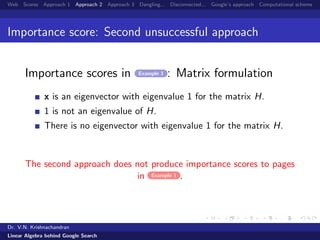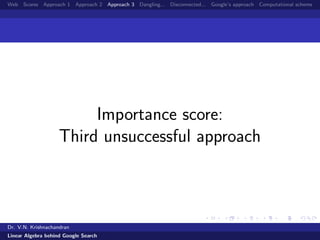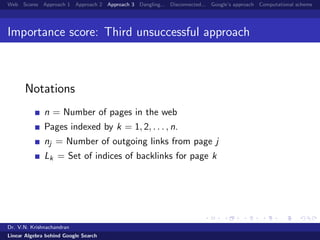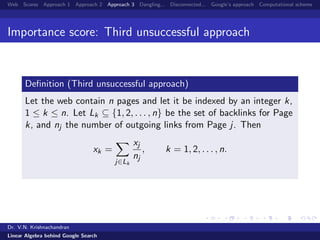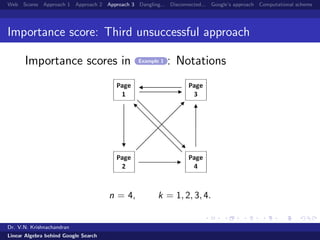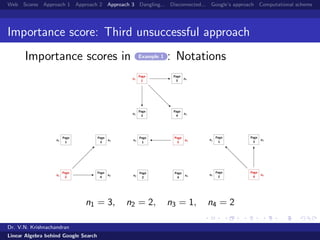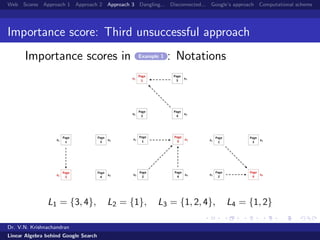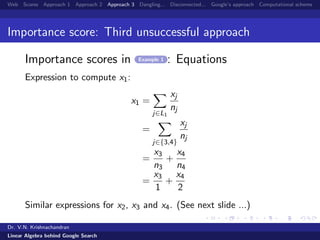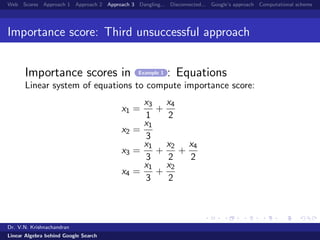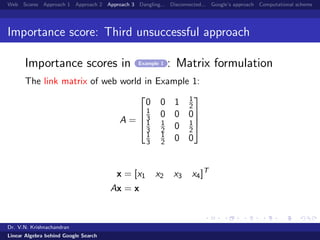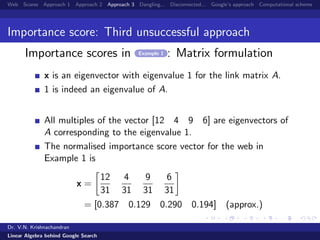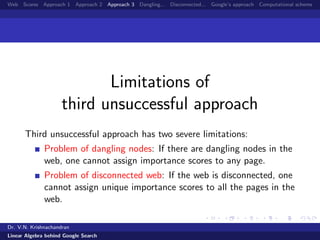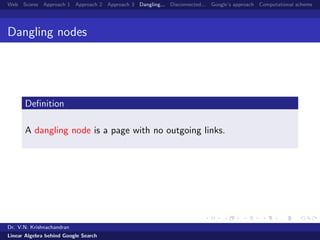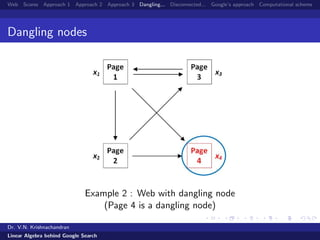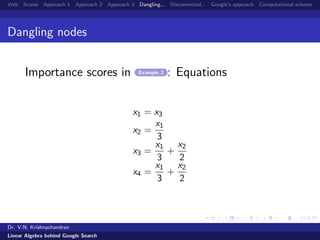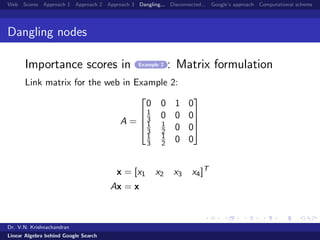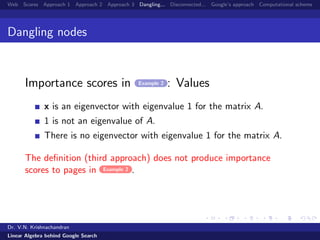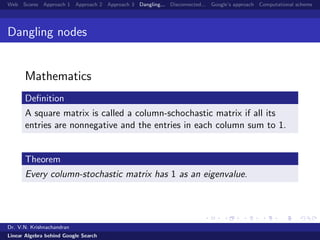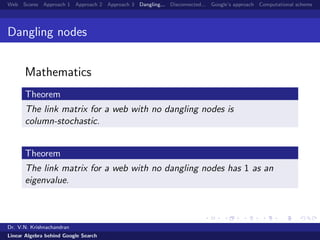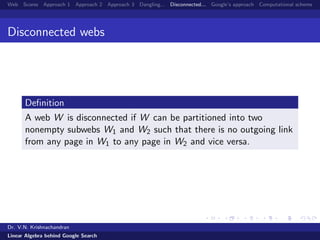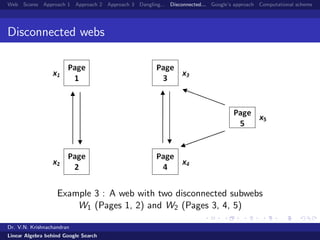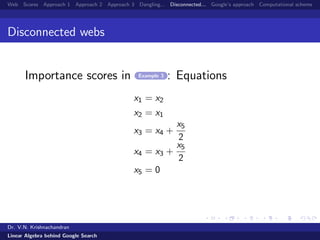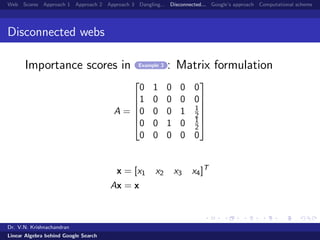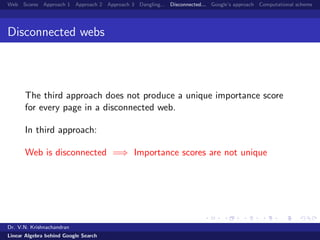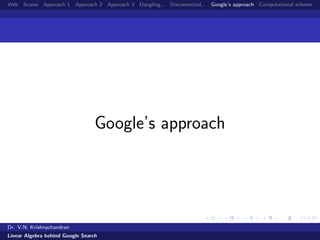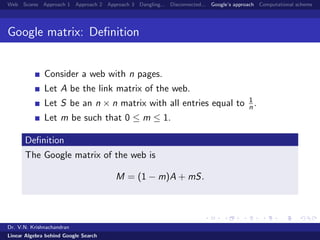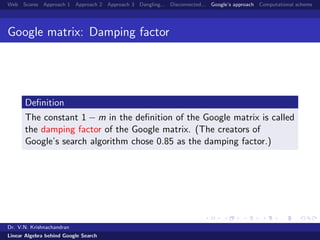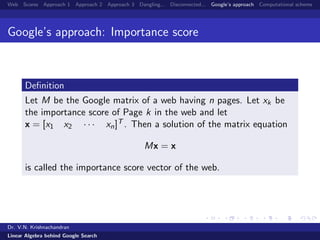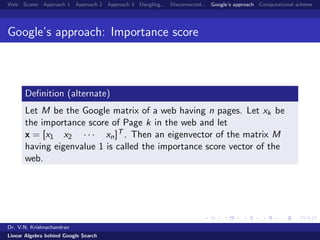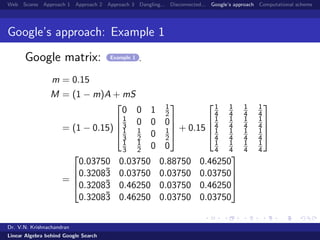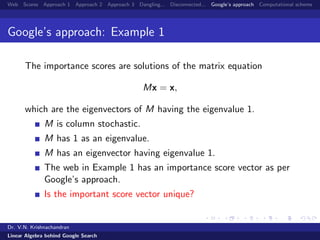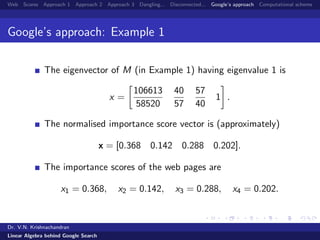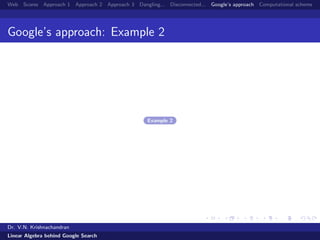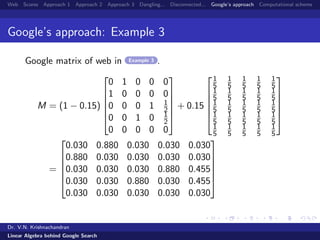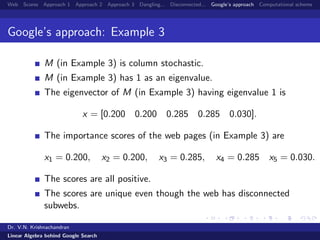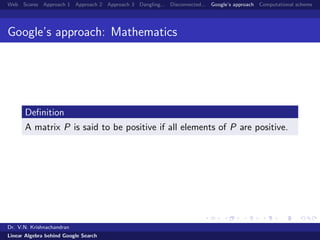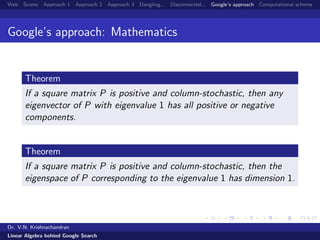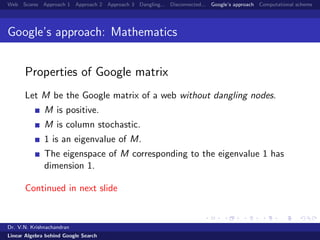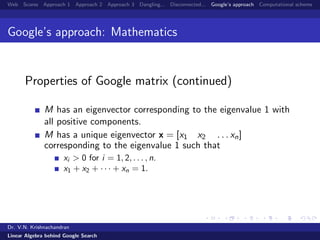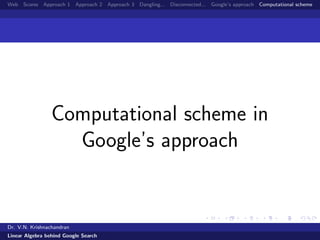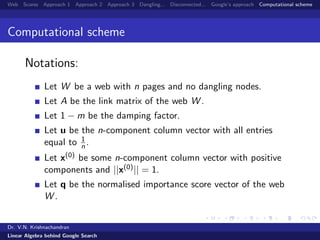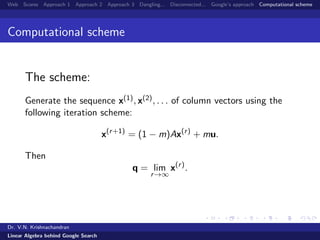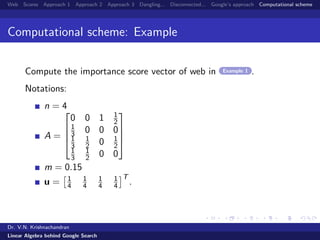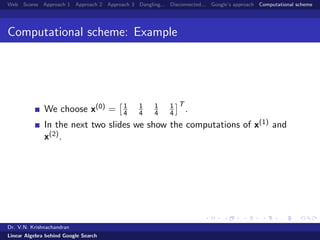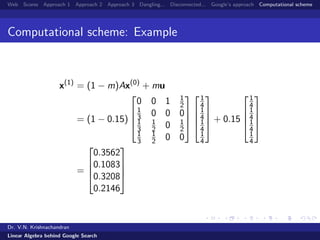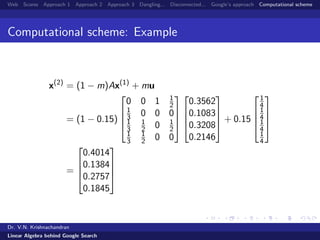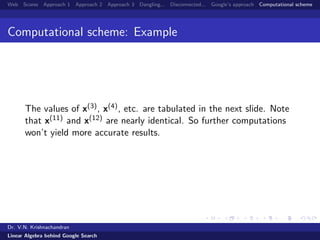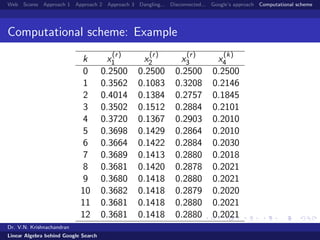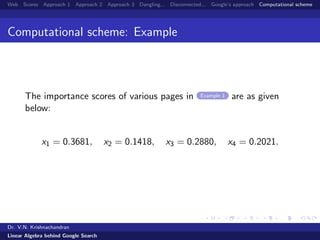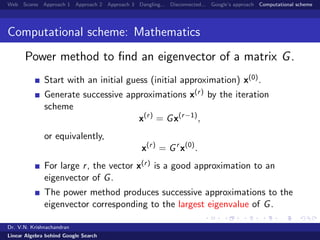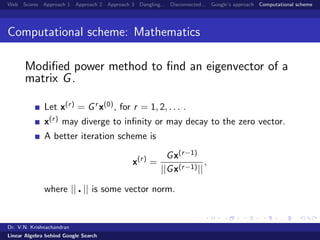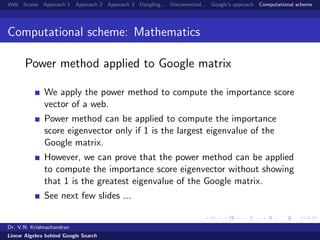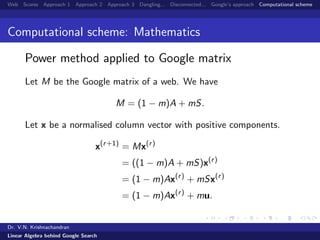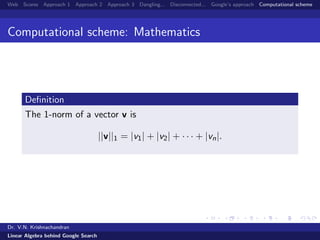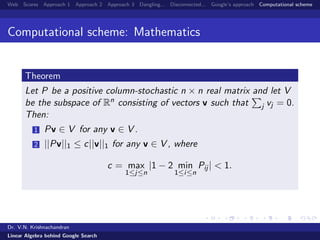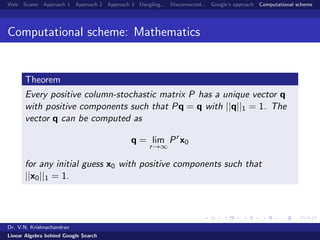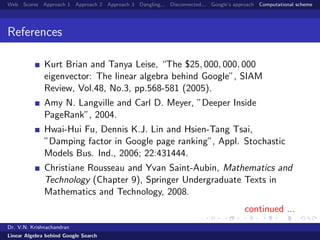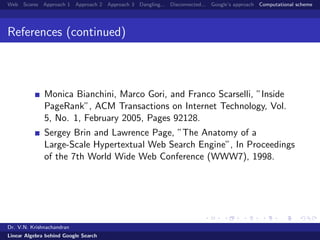1 von 80

### Linear algebra behind Google search

• 1. Web Scores Approach 1 Approach 2 Approach 3 Dangling... Disconnected... Google’s approach Computational scheme Linear Algebra behind Google Search Dr. V.N. Krishnachandran Department of Computer Applications Vidya Academy of Science and Technology Thrissur - 680501, Kerala. August 2011 Dr. V.N. Krishnachandran Linear Algebra behind Google Search
• 2. Web Scores Approach 1 Approach 2 Approach 3 Dangling... Disconnected... Google’s approach Computational scheme Outline 1 Web: An example 2 Importance score 3 First unsuccessful approach 4 Second unsuccessful approach 5 Third unsuccessful approach 6 Dangling nodes 7 Disconnected webs 8 Google approach 9 Computational scheme Dr. V.N. Krishnachandran Linear Algebra behind Google Search
• 3. Web Scores Approach 1 Approach 2 Approach 3 Dangling... Disconnected... Google’s approach Computational scheme Web world The web world consists of a number of pages and links from some of the pages to some other pages. In a diagrammatic representation of a web world, pages are denoted by small squares or circles and links are indicated by arrows. See a simpliﬁed web world in next slide. Dr. V.N. Krishnachandran Linear Algebra behind Google Search
• 4. Web Scores Approach 1 Approach 2 Approach 3 Dangling... Disconnected... Google’s approach Computational scheme Web world Example 1: A web with four pages numbered 1,2,3,4. Dr. V.N. Krishnachandran Linear Algebra behind Google Search
• 5. Web Scores Approach 1 Approach 2 Approach 3 Dangling... Disconnected... Google’s approach Computational scheme Links In the ﬁgure above, arrow denotes: an incoming link (also called a backlink) to Page q. an outgoing link from Page p. Dr. V.N. Krishnachandran Linear Algebra behind Google Search
• 6. Web Scores Approach 1 Approach 2 Approach 3 Dangling... Disconnected... Google’s approach Computational scheme Links Outgoing links in Example 1 Dr. V.N. Krishnachandran Linear Algebra behind Google Search
• 7. Web Scores Approach 1 Approach 2 Approach 3 Dangling... Disconnected... Google’s approach Computational scheme Links Incoming links in Example 1 Dr. V.N. Krishnachandran Linear Algebra behind Google Search
• 8. Web Scores Approach 1 Approach 2 Approach 3 Dangling... Disconnected... Google’s approach Computational scheme Importance score In Google’s search algorithm, the most important concept is that of the importance score of a page. This we explain in the next few slides... Dr. V.N. Krishnachandran Linear Algebra behind Google Search
• 9. Web Scores Approach 1 Approach 2 Approach 3 Dangling... Disconnected... Google’s approach Computational scheme Importance score The importance score, or simply the score, of a page is a number which is a measure of the relative importance of a page. The importance score is a nonnegative real number. The importance score of a page is derived from the backlinks for that page. Dr. V.N. Krishnachandran Linear Algebra behind Google Search
• 10. Web Scores Approach 1 Approach 2 Approach 3 Dangling... Disconnected... Google’s approach Computational scheme Importance score vector We denote the importance score of Page k by xk. Let there be n pages in the web. The column vector x = [x1 x2 · · · xn]T is called the importance score vector. The importance score vector x is said to be normalised if x1 + x2 + · · · xn = 1. Dr. V.N. Krishnachandran Linear Algebra behind Google Search
• 11. Web Scores Approach 1 Approach 2 Approach 3 Dangling... Disconnected... Google’s approach Computational scheme Unsuccessful attempts to deﬁne importance score Before considering Google’s approach, we consider three unsuccessful attempts to deﬁne the concept of the importance score of a page. A study of these unsuccessful attempts helps one appreciate the signiﬁcance of Google’s approach. Dr. V.N. Krishnachandran Linear Algebra behind Google Search
• 12. Web Scores Approach 1 Approach 2 Approach 3 Dangling... Disconnected... Google’s approach Computational scheme Importance score: First unsuccessful approach Dr. V.N. Krishnachandran Linear Algebra behind Google Search
• 13. Web Scores Approach 1 Approach 2 Approach 3 Dangling... Disconnected... Google’s approach Computational scheme Importance score: First unsuccessful approach Deﬁnition (First unsuccessful approach) Importance score of Page k is the number of backlinks for Page k. Dr. V.N. Krishnachandran Linear Algebra behind Google Search
• 14. Web Scores Approach 1 Approach 2 Approach 3 Dangling... Disconnected... Google’s approach Computational scheme Importance score: First unsuccessful approach Importance scores in Example 1 Dr. V.N. Krishnachandran Linear Algebra behind Google Search
• 15. Web Scores Approach 1 Approach 2 Approach 3 Dangling... Disconnected... Google’s approach Computational scheme Importance score Importance score: A desirable property “A link to Page k from an important page must increase Page k’s score more than a link from an unimportant page.” First unsuccessful approach does not have this property. (see next slide) Dr. V.N. Krishnachandran Linear Algebra behind Google Search
• 16. Web Scores Approach 1 Approach 2 Approach 3 Dangling... Disconnected... Google’s approach Computational scheme Importance score: First unsuccessful approach Importance score of Page 1 must be higher than that of Page 4. Dr. V.N. Krishnachandran Linear Algebra behind Google Search
• 17. Web Scores Approach 1 Approach 2 Approach 3 Dangling... Disconnected... Google’s approach Computational scheme Importance score: Second unsuccessful approach Dr. V.N. Krishnachandran Linear Algebra behind Google Search
• 18. Web Scores Approach 1 Approach 2 Approach 3 Dangling... Disconnected... Google’s approach Computational scheme Importance score: Second unsuccessful approach Deﬁnition (Second unsuccessful approach) The importance score of a page is the sum of the scores of all pages linking to the page. Dr. V.N. Krishnachandran Linear Algebra behind Google Search
• 19. Web Scores Approach 1 Approach 2 Approach 3 Dangling... Disconnected... Google’s approach Computational scheme Importance score: Second unsuccessful approach Importance scores in Example 1 The importance scores in Example 1 (second approach) are solutions of the following system of equations: x1 = x3 + x4 x2 = x1 x3 = x1 + x2 + x4 x4 = x1 + x2 Dr. V.N. Krishnachandran Linear Algebra behind Google Search
• 20. Web Scores Approach 1 Approach 2 Approach 3 Dangling... Disconnected... Google’s approach Computational scheme Importance score: Second unsuccessful approach Importance scores in Example 1 : Matrix formulation H =     0 0 1 1 1 0 0 0 1 1 0 1 1 1 0 0     x = [x1 x2 x3 x4]T Hx = x Dr. V.N. Krishnachandran Linear Algebra behind Google Search
• 21. Web Scores Approach 1 Approach 2 Approach 3 Dangling... Disconnected... Google’s approach Computational scheme Importance score: Second unsuccessful approach Importance scores in Example 1 : Matrix formulation x is an eigenvector with eigenvalue 1 for the matrix H. 1 is not an eigenvalue of H. There is no eigenvector with eigenvalue 1 for the matrix H. The second approach does not produce importance scores to pages in Example 1 . Dr. V.N. Krishnachandran Linear Algebra behind Google Search
• 22. Web Scores Approach 1 Approach 2 Approach 3 Dangling... Disconnected... Google’s approach Computational scheme Importance score: Second unsuccessful approach Importance score: An undesirable property “A page with many outgoing links has a bigger inﬂuence on the scores of other pages than a page with less number of outgoing links.” This is undesirable. The recommendation letter of a Professor who is choosy in giving such letters carries higher value than that of a Professor who is very liberal in issuing such letters. Dr. V.N. Krishnachandran Linear Algebra behind Google Search
• 23. Web Scores Approach 1 Approach 2 Approach 3 Dangling... Disconnected... Google’s approach Computational scheme Importance score: Third unsuccessful approach Dr. V.N. Krishnachandran Linear Algebra behind Google Search
• 24. Web Scores Approach 1 Approach 2 Approach 3 Dangling... Disconnected... Google’s approach Computational scheme Importance score: Third unsuccessful approach Notations n = Number of pages in the web Pages indexed by k = 1, 2, . . . , n. nj = Number of outgoing links from page j Lk = Set of indices of backlinks for page k Dr. V.N. Krishnachandran Linear Algebra behind Google Search
• 25. Web Scores Approach 1 Approach 2 Approach 3 Dangling... Disconnected... Google’s approach Computational scheme Importance score: Third unsuccessful approach Deﬁnition (Third unsuccessful approach) Let the web contain n pages and let it be indexed by an integer k, 1 ≤ k ≤ n. Let Lk ⊆ {1, 2, . . . , n} be the set of backlinks for Page k, and nj the number of outgoing links from Page j. Then xk = j∈Lk xj nj , k = 1, 2, . . . , n. Dr. V.N. Krishnachandran Linear Algebra behind Google Search
• 26. Web Scores Approach 1 Approach 2 Approach 3 Dangling... Disconnected... Google’s approach Computational scheme Importance score: Third unsuccessful approach Importance scores in Example 1 : Notations n = 4, k = 1, 2, 3, 4. Dr. V.N. Krishnachandran Linear Algebra behind Google Search
• 27. Web Scores Approach 1 Approach 2 Approach 3 Dangling... Disconnected... Google’s approach Computational scheme Importance score: Third unsuccessful approach Importance scores in Example 1 : Notations n1 = 3, n2 = 2, n3 = 1, n4 = 2 Dr. V.N. Krishnachandran Linear Algebra behind Google Search
• 28. Web Scores Approach 1 Approach 2 Approach 3 Dangling... Disconnected... Google’s approach Computational scheme Importance score: Third unsuccessful approach Importance scores in Example 1 : Notations L1 = {3, 4}, L2 = {1}, L3 = {1, 2, 4}, L4 = {1, 2} Dr. V.N. Krishnachandran Linear Algebra behind Google Search
• 29. Web Scores Approach 1 Approach 2 Approach 3 Dangling... Disconnected... Google’s approach Computational scheme Importance score: Third unsuccessful approach Importance scores in Example 1 : Equations Expression to compute x1: x1 = j∈L1 xj nj = j∈{3,4} xj nj = x3 n3 + x4 n4 = x3 1 + x4 2 Similar expressions for x2, x3 and x4. (See next slide ...) Dr. V.N. Krishnachandran Linear Algebra behind Google Search
• 30. Web Scores Approach 1 Approach 2 Approach 3 Dangling... Disconnected... Google’s approach Computational scheme Importance score: Third unsuccessful approach Importance scores in Example 1 : Equations Linear system of equations to compute importance score: x1 = x3 1 + x4 2 x2 = x1 3 x3 = x1 3 + x2 2 + x4 2 x4 = x1 3 + x2 2 Dr. V.N. Krishnachandran Linear Algebra behind Google Search
• 31. Web Scores Approach 1 Approach 2 Approach 3 Dangling... Disconnected... Google’s approach Computational scheme Importance score: Third unsuccessful approach Importance scores in Example 1 : Matrix formulation The link matrix of web world in Example 1: A =     0 0 1 1 2 1 3 0 0 0 1 3 1 2 0 1 2 1 3 1 2 0 0     x = [x1 x2 x3 x4]T Ax = x Dr. V.N. Krishnachandran Linear Algebra behind Google Search
• 32. Web Scores Approach 1 Approach 2 Approach 3 Dangling... Disconnected... Google’s approach Computational scheme Importance score: Third unsuccessful approach Importance scores in Example 1 : Matrix formulation x is an eigenvector with eigenvalue 1 for the link matrix A. 1 is indeed an eigenvalue of A. All multiples of the vector [12 4 9 6] are eigenvectors of A corresponding to the eigenvalue 1. The normalised importance score vector for the web in Example 1 is x = 12 31 4 31 9 31 6 31 = [0.387 0.129 0.290 0.194] (approx.) Dr. V.N. Krishnachandran Linear Algebra behind Google Search
• 33. Web Scores Approach 1 Approach 2 Approach 3 Dangling... Disconnected... Google’s approach Computational scheme Limitations of third unsuccessful approach Third unsuccessful approach has two severe limitations: Problem of dangling nodes: If there are dangling nodes in the web, one cannot assign importance scores to any page. Problem of disconnected web: If the web is disconnected, one cannot assign unique importance scores to all the pages in the web. Dr. V.N. Krishnachandran Linear Algebra behind Google Search
• 34. Web Scores Approach 1 Approach 2 Approach 3 Dangling... Disconnected... Google’s approach Computational scheme Dangling nodes Deﬁnition A dangling node is a page with no outgoing links. Dr. V.N. Krishnachandran Linear Algebra behind Google Search
• 35. Web Scores Approach 1 Approach 2 Approach 3 Dangling... Disconnected... Google’s approach Computational scheme Dangling nodes Example 2 : Web with dangling node (Page 4 is a dangling node) Dr. V.N. Krishnachandran Linear Algebra behind Google Search
• 36. Web Scores Approach 1 Approach 2 Approach 3 Dangling... Disconnected... Google’s approach Computational scheme Dangling nodes Importance scores in Example 2 : Equations x1 = x3 x2 = x1 3 x3 = x1 3 + x2 2 x4 = x1 3 + x2 2 Dr. V.N. Krishnachandran Linear Algebra behind Google Search
• 37. Web Scores Approach 1 Approach 2 Approach 3 Dangling... Disconnected... Google’s approach Computational scheme Dangling nodes Importance scores in Example 2 : Matrix formulation Link matrix for the web in Example 2: A =     0 0 1 0 1 3 0 0 0 1 3 1 2 0 0 1 3 1 2 0 0     x = [x1 x2 x3 x4]T Ax = x Dr. V.N. Krishnachandran Linear Algebra behind Google Search
• 38. Web Scores Approach 1 Approach 2 Approach 3 Dangling... Disconnected... Google’s approach Computational scheme Dangling nodes Importance scores in Example 2 : Values x is an eigenvector with eigenvalue 1 for the matrix A. 1 is not an eigenvalue of A. There is no eigenvector with eigenvalue 1 for the matrix A. The deﬁnition (third approach) does not produce importance scores to pages in Example 2 . Dr. V.N. Krishnachandran Linear Algebra behind Google Search
• 39. Web Scores Approach 1 Approach 2 Approach 3 Dangling... Disconnected... Google’s approach Computational scheme Dangling nodes Mathematics Deﬁnition A square matrix is called a column-schochastic matrix if all its entries are nonnegative and the entries in each column sum to 1. Theorem Every column-stochastic matrix has 1 as an eigenvalue. Dr. V.N. Krishnachandran Linear Algebra behind Google Search
• 40. Web Scores Approach 1 Approach 2 Approach 3 Dangling... Disconnected... Google’s approach Computational scheme Dangling nodes Mathematics Theorem The link matrix for a web with no dangling nodes is column-stochastic. Theorem The link matrix for a web with no dangling nodes has 1 as an eigenvalue. Dr. V.N. Krishnachandran Linear Algebra behind Google Search
• 41. Web Scores Approach 1 Approach 2 Approach 3 Dangling... Disconnected... Google’s approach Computational scheme Disconnected webs Deﬁnition A web W is disconnected if W can be partitioned into two nonempty subwebs W1 and W2 such that there is no outgoing link from any page in W1 to any page in W2 and vice versa. Dr. V.N. Krishnachandran Linear Algebra behind Google Search
• 42. Web Scores Approach 1 Approach 2 Approach 3 Dangling... Disconnected... Google’s approach Computational scheme Disconnected webs Example 3 : A web with two disconnected subwebs W1 (Pages 1, 2) and W2 (Pages 3, 4, 5) Dr. V.N. Krishnachandran Linear Algebra behind Google Search
• 43. Web Scores Approach 1 Approach 2 Approach 3 Dangling... Disconnected... Google’s approach Computational scheme Disconnected webs Importance scores in Example 3 : Equations x1 = x2 x2 = x1 x3 = x4 + x5 2 x4 = x3 + x5 2 x5 = 0 Dr. V.N. Krishnachandran Linear Algebra behind Google Search
• 44. Web Scores Approach 1 Approach 2 Approach 3 Dangling... Disconnected... Google’s approach Computational scheme Disconnected webs Importance scores in Example 3 : Matrix formulation A =       0 1 0 0 0 1 0 0 0 0 0 0 0 1 1 2 0 0 1 0 1 2 0 0 0 0 0       x = [x1 x2 x3 x4]T Ax = x Dr. V.N. Krishnachandran Linear Algebra behind Google Search
• 45. Web Scores Approach 1 Approach 2 Approach 3 Dangling... Disconnected... Google’s approach Computational scheme Disconnected webs Importance scores in Example 3 : Values Two linearly independent eigenvectors with eigenvalue 1: x = 1 2 1 2 0 0 0 x = 0 0 1 2 1 2 0 These are linearly independent, normalised, importance score vectors in Example 3 . Dr. V.N. Krishnachandran Linear Algebra behind Google Search
• 46. Web Scores Approach 1 Approach 2 Approach 3 Dangling... Disconnected... Google’s approach Computational scheme Disconnected webs The third approach does not produce a unique importance score for every page in a disconnected web. In third approach: Web is disconnected =⇒ Importance scores are not unique Dr. V.N. Krishnachandran Linear Algebra behind Google Search
• 47. Web Scores Approach 1 Approach 2 Approach 3 Dangling... Disconnected... Google’s approach Computational scheme Google’s approach Dr. V.N. Krishnachandran Linear Algebra behind Google Search
• 48. Web Scores Approach 1 Approach 2 Approach 3 Dangling... Disconnected... Google’s approach Computational scheme Google matrix: Deﬁnition Consider a web with n pages. Let A be the link matrix of the web. Let S be an n × n matrix with all entries equal to 1 n . Let m be such that 0 ≤ m ≤ 1. Deﬁnition The Google matrix of the web is M = (1 − m)A + mS. Dr. V.N. Krishnachandran Linear Algebra behind Google Search
• 49. Web Scores Approach 1 Approach 2 Approach 3 Dangling... Disconnected... Google’s approach Computational scheme Google matrix: Damping factor Deﬁnition The constant 1 − m in the deﬁnition of the Google matrix is called the damping factor of the Google matrix. (The creators of Google’s search algorithm chose 0.85 as the damping factor.) Dr. V.N. Krishnachandran Linear Algebra behind Google Search
• 50. Web Scores Approach 1 Approach 2 Approach 3 Dangling... Disconnected... Google’s approach Computational scheme Google’s approach: Importance score Deﬁnition Let M be the Google matrix of a web having n pages. Let xk be the importance score of Page k in the web and let x = [x1 x2 · · · xn]T . Then a solution of the matrix equation Mx = x is called the importance score vector of the web. Dr. V.N. Krishnachandran Linear Algebra behind Google Search
• 51. Web Scores Approach 1 Approach 2 Approach 3 Dangling... Disconnected... Google’s approach Computational scheme Google’s approach: Importance score Deﬁnition (alternate) Let M be the Google matrix of a web having n pages. Let xk be the importance score of Page k in the web and let x = [x1 x2 · · · xn]T . Then an eigenvector of the matrix M having eigenvalue 1 is called the importance score vector of the web. Dr. V.N. Krishnachandran Linear Algebra behind Google Search
• 52. Web Scores Approach 1 Approach 2 Approach 3 Dangling... Disconnected... Google’s approach Computational scheme Google’s approach: Example 1 Google matrix: Example 1 . m = 0.15 M = (1 − m)A + mS = (1 − 0.15)     0 0 1 1 2 1 3 0 0 0 1 3 1 2 0 1 2 1 3 1 2 0 0     + 0.15     1 4 1 4 1 4 1 4 1 4 1 4 1 4 1 4 1 4 1 4 1 4 1 4 1 4 1 4 1 4 1 4     =     0.03750 0.03750 0.88750 0.46250 0.3208¯3 0.03750 0.03750 0.03750 0.3208¯3 0.46250 0.03750 0.46250 0.3208¯3 0.46250 0.03750 0.03750     Dr. V.N. Krishnachandran Linear Algebra behind Google Search
• 53. Web Scores Approach 1 Approach 2 Approach 3 Dangling... Disconnected... Google’s approach Computational scheme Google’s approach: Example 1 The importance scores are solutions of the matrix equation Mx = x, which are the eigenvectors of M having the eigenvalue 1. M is column stochastic. M has 1 as an eigenvalue. M has an eigenvector having eigenvalue 1. The web in Example 1 has an importance score vector as per Google’s approach. Is the important score vector unique? Dr. V.N. Krishnachandran Linear Algebra behind Google Search
• 54. Web Scores Approach 1 Approach 2 Approach 3 Dangling... Disconnected... Google’s approach Computational scheme Google’s approach: Example 1 The eigenvector of M (in Example 1) having eigenvalue 1 is x = 106613 58520 40 57 57 40 1 . The normalised importance score vector is (approximately) x = [0.368 0.142 0.288 0.202]. The importance scores of the web pages are x1 = 0.368, x2 = 0.142, x3 = 0.288, x4 = 0.202. Dr. V.N. Krishnachandran Linear Algebra behind Google Search
• 55. Web Scores Approach 1 Approach 2 Approach 3 Dangling... Disconnected... Google’s approach Computational scheme Google’s approach: Example 2 Example 2 Dr. V.N. Krishnachandran Linear Algebra behind Google Search
• 56. Web Scores Approach 1 Approach 2 Approach 3 Dangling... Disconnected... Google’s approach Computational scheme Google’s approach: Example 3 Google matrix of web in Example 3 . M = (1 − 0.15)       0 1 0 0 0 1 0 0 0 0 0 0 0 1 1 2 0 0 1 0 1 2 0 0 0 0 0       + 0.15       1 5 1 5 1 5 1 5 1 5 1 5 1 5 1 5 1 5 1 5 1 5 1 5 1 5 1 5 1 5 1 5 1 5 1 5 1 5 1 5 1 5 1 5 1 5 1 5 1 5       =       0.030 0.880 0.030 0.030 0.030 0.880 0.030 0.030 0.030 0.030 0.030 0.030 0.030 0.880 0.455 0.030 0.030 0.880 0.030 0.455 0.030 0.030 0.030 0.030 0.030       Dr. V.N. Krishnachandran Linear Algebra behind Google Search
• 57. Web Scores Approach 1 Approach 2 Approach 3 Dangling... Disconnected... Google’s approach Computational scheme Google’s approach: Example 3 M (in Example 3) is column stochastic. M (in Example 3) has 1 as an eigenvalue. The eigenvector of M (in Example 3) having eigenvalue 1 is x = [0.200 0.200 0.285 0.285 0.030]. The importance scores of the web pages (in Example 3) are x1 = 0.200, x2 = 0.200, x3 = 0.285, x4 = 0.285 x5 = 0.030. The scores are all positive. The scores are unique even though the web has disconnected subwebs. Dr. V.N. Krishnachandran Linear Algebra behind Google Search
• 58. Web Scores Approach 1 Approach 2 Approach 3 Dangling... Disconnected... Google’s approach Computational scheme Google’s approach: Mathematics Deﬁnition A matrix P is said to be positive if all elements of P are positive. Dr. V.N. Krishnachandran Linear Algebra behind Google Search
• 59. Web Scores Approach 1 Approach 2 Approach 3 Dangling... Disconnected... Google’s approach Computational scheme Google’s approach: Mathematics Theorem If a square matrix P is positive and column-stochastic, then any eigenvector of P with eigenvalue 1 has all positive or negative components. Theorem If a square matrix P is positive and column-stochastic, then the eigenspace of P corresponding to the eigenvalue 1 has dimension 1. Dr. V.N. Krishnachandran Linear Algebra behind Google Search
• 60. Web Scores Approach 1 Approach 2 Approach 3 Dangling... Disconnected... Google’s approach Computational scheme Google’s approach: Mathematics Properties of Google matrix Let M be the Google matrix of a web without dangling nodes. M is positive. M is column stochastic. 1 is an eigenvalue of M. The eigenspace of M corresponding to the eigenvalue 1 has dimension 1. Continued in next slide Dr. V.N. Krishnachandran Linear Algebra behind Google Search
• 61. Web Scores Approach 1 Approach 2 Approach 3 Dangling... Disconnected... Google’s approach Computational scheme Google’s approach: Mathematics Properties of Google matrix (continued) M has an eigenvector corresponding to the eigenvalue 1 with all positive components. M has a unique eigenvector x = [x1 x2 . . . xn] corresponding to the eigenvalue 1 such that xi > 0 for i = 1, 2, . . . , n. x1 + x2 + · · · + xn = 1. Dr. V.N. Krishnachandran Linear Algebra behind Google Search
• 62. Web Scores Approach 1 Approach 2 Approach 3 Dangling... Disconnected... Google’s approach Computational scheme Computational scheme in Google’s approach Dr. V.N. Krishnachandran Linear Algebra behind Google Search
• 63. Web Scores Approach 1 Approach 2 Approach 3 Dangling... Disconnected... Google’s approach Computational scheme Computational scheme Notations: Let W be a web with n pages and no dangling nodes. Let A be the link matrix of the web W . Let 1 − m be the damping factor. Let u be the n-component column vector with all entries equal to 1 n . Let x(0) be some n-component column vector with positive components and ||x(0)|| = 1. Let q be the normalised importance score vector of the web W . Dr. V.N. Krishnachandran Linear Algebra behind Google Search
• 64. Web Scores Approach 1 Approach 2 Approach 3 Dangling... Disconnected... Google’s approach Computational scheme Computational scheme The scheme: Generate the sequence x(1), x(2), . . . of column vectors using the following iteration scheme: x(r+1) = (1 − m)Ax(r) + mu. Then q = lim r→∞ x(r) . Dr. V.N. Krishnachandran Linear Algebra behind Google Search
• 65. Web Scores Approach 1 Approach 2 Approach 3 Dangling... Disconnected... Google’s approach Computational scheme Computational scheme: Example Compute the importance score vector of web in Example 1 . Notations: n = 4 A =     0 0 1 1 2 1 3 0 0 0 1 3 1 2 0 1 2 1 3 1 2 0 0     m = 0.15 u = 1 4 1 4 1 4 1 4 T . Dr. V.N. Krishnachandran Linear Algebra behind Google Search
• 66. Web Scores Approach 1 Approach 2 Approach 3 Dangling... Disconnected... Google’s approach Computational scheme Computational scheme: Example We choose x(0) = 1 4 1 4 1 4 1 4 T . In the next two slides we show the computations of x(1) and x(2). Dr. V.N. Krishnachandran Linear Algebra behind Google Search
• 67. Web Scores Approach 1 Approach 2 Approach 3 Dangling... Disconnected... Google’s approach Computational scheme Computational scheme: Example x(1) = (1 − m)Ax(0) + mu = (1 − 0.15)     0 0 1 1 2 1 3 0 0 0 1 3 1 2 0 1 2 1 3 1 2 0 0         1 4 1 4 1 4 1 4     + 0.15     1 4 1 4 1 4 1 4     =     0.3562 0.1083 0.3208 0.2146     Dr. V.N. Krishnachandran Linear Algebra behind Google Search
• 68. Web Scores Approach 1 Approach 2 Approach 3 Dangling... Disconnected... Google’s approach Computational scheme Computational scheme: Example x(2) = (1 − m)Ax(1) + mu = (1 − 0.15)     0 0 1 1 2 1 3 0 0 0 1 3 1 2 0 1 2 1 3 1 2 0 0         0.3562 0.1083 0.3208 0.2146     + 0.15     1 4 1 4 1 4 1 4     =     0.4014 0.1384 0.2757 0.1845     Dr. V.N. Krishnachandran Linear Algebra behind Google Search
• 69. Web Scores Approach 1 Approach 2 Approach 3 Dangling... Disconnected... Google’s approach Computational scheme Computational scheme: Example The values of x(3), x(4), etc. are tabulated in the next slide. Note that x(11) and x(12) are nearly identical. So further computations won’t yield more accurate results. Dr. V.N. Krishnachandran Linear Algebra behind Google Search
• 70. Web Scores Approach 1 Approach 2 Approach 3 Dangling... Disconnected... Google’s approach Computational scheme Computational scheme: Example k x (r) 1 x (r) 2 x (r) 3 x (k) 4 0 0.2500 0.2500 0.2500 0.2500 1 0.3562 0.1083 0.3208 0.2146 2 0.4014 0.1384 0.2757 0.1845 3 0.3502 0.1512 0.2884 0.2101 4 0.3720 0.1367 0.2903 0.2010 5 0.3698 0.1429 0.2864 0.2010 6 0.3664 0.1422 0.2884 0.2030 7 0.3689 0.1413 0.2880 0.2018 8 0.3681 0.1420 0.2878 0.2021 9 0.3680 0.1418 0.2880 0.2021 10 0.3682 0.1418 0.2879 0.2020 11 0.3681 0.1418 0.2880 0.2021 12 0.3681 0.1418 0.2880 0.2021 Dr. V.N. Krishnachandran Linear Algebra behind Google Search
• 71. Web Scores Approach 1 Approach 2 Approach 3 Dangling... Disconnected... Google’s approach Computational scheme Computational scheme: Example The importance scores of various pages in Example 1 are as given below: x1 = 0.3681, x2 = 0.1418, x3 = 0.2880, x4 = 0.2021. Dr. V.N. Krishnachandran Linear Algebra behind Google Search
• 72. Web Scores Approach 1 Approach 2 Approach 3 Dangling... Disconnected... Google’s approach Computational scheme Computational scheme: Mathematics Power method to ﬁnd an eigenvector of a matrix G. Start with an initial guess (initial approximation) x(0). Generate successive approximations x(r) by the iteration scheme x(r) = Gx(r−1) , or equivalently, x(r) = Gr x(0) . For large r, the vector x(r) is a good approximation to an eigenvector of G. The power method produces successive approximations to the eigenvector corresponding to the largest eigenvalue of G. Dr. V.N. Krishnachandran Linear Algebra behind Google Search
• 73. Web Scores Approach 1 Approach 2 Approach 3 Dangling... Disconnected... Google’s approach Computational scheme Computational scheme: Mathematics Modiﬁed power method to ﬁnd an eigenvector of a matrix G. Let x(r) = Gr x(0), for r = 1, 2, . . . . x(r) may diverge to inﬁnity or may decay to the zero vector. A better iteration scheme is x(r) = Gx(r−1) ||Gx(r−1)|| , where || || is some vector norm. Dr. V.N. Krishnachandran Linear Algebra behind Google Search
• 74. Web Scores Approach 1 Approach 2 Approach 3 Dangling... Disconnected... Google’s approach Computational scheme Computational scheme: Mathematics Power method applied to Google matrix We apply the power method to compute the importance score vector of a web. Power method can be applied to compute the importance score eigenvector only if 1 is the largest eigenvalue of the Google matrix. However, we can prove that the power method can be applied to compute the importance score eigenvector without showing that 1 is the greatest eigenvalue of the Google matrix. See next few slides ... Dr. V.N. Krishnachandran Linear Algebra behind Google Search
• 75. Web Scores Approach 1 Approach 2 Approach 3 Dangling... Disconnected... Google’s approach Computational scheme Computational scheme: Mathematics Power method applied to Google matrix Let M be the Google matrix of a web. We have M = (1 − m)A + mS. Let x be a normalised column vector with positive components. x(r+1) = Mx(r) = ((1 − m)A + mS)x(r) = (1 − m)Ax(r) + mSx(r) = (1 − m)Ax(r) + mu. Dr. V.N. Krishnachandran Linear Algebra behind Google Search
• 76. Web Scores Approach 1 Approach 2 Approach 3 Dangling... Disconnected... Google’s approach Computational scheme Computational scheme: Mathematics Deﬁnition The 1-norm of a vector v is ||v||1 = |v1| + |v2| + · · · + |vn|. Dr. V.N. Krishnachandran Linear Algebra behind Google Search
• 77. Web Scores Approach 1 Approach 2 Approach 3 Dangling... Disconnected... Google’s approach Computational scheme Computational scheme: Mathematics Theorem Let P be a positive column-stochastic n × n real matrix and let V be the subspace of Rn consisting of vectors v such that j vj = 0. Then: 1 Pv ∈ V for any v ∈ V . 2 ||Pv||1 ≤ c||v||1 for any v ∈ V , where c = max 1≤j≤n |1 − 2 min 1≤i≤n Pij | < 1. Dr. V.N. Krishnachandran Linear Algebra behind Google Search
• 78. Web Scores Approach 1 Approach 2 Approach 3 Dangling... Disconnected... Google’s approach Computational scheme Computational scheme: Mathematics Theorem Every positive column-stochastic matrix P has a unique vector q with positive components such that Pq = q with ||q||1 = 1. The vector q can be computed as q = lim r→∞ Pr x0 for any initial guess x0 with positive components such that ||x0||1 = 1. Dr. V.N. Krishnachandran Linear Algebra behind Google Search
• 79. Web Scores Approach 1 Approach 2 Approach 3 Dangling... Disconnected... Google’s approach Computational scheme References Kurt Brian and Tanya Leise, “The \$25, 000, 000, 000 eigenvector: The linear algebra behind Google”, SIAM Review, Vol.48, No.3, pp.568-581 (2005). Amy N. Langville and Carl D. Meyer, ”Deeper Inside PageRank”, 2004. Hwai-Hui Fu, Dennis K.J. Lin and Hsien-Tang Tsai, ”Damping factor in Google page ranking”, Appl. Stochastic Models Bus. Ind., 2006; 22:431444. Christiane Rousseau and Yvan Saint-Aubin, Mathematics and Technology (Chapter 9), Springer Undergraduate Texts in Mathematics and Technology, 2008. continued ... Dr. V.N. Krishnachandran Linear Algebra behind Google Search
• 80. Web Scores Approach 1 Approach 2 Approach 3 Dangling... Disconnected... Google’s approach Computational scheme References (continued) Monica Bianchini, Marco Gori, and Franco Scarselli, ”Inside PageRank”, ACM Transactions on Internet Technology, Vol. 5, No. 1, February 2005, Pages 92128. Sergey Brin and Lawrence Page, ”The Anatomy of a Large-Scale Hypertextual Web Search Engine”, In Proceedings of the 7th World Wide Web Conference (WWW7), 1998. Dr. V.N. Krishnachandran Linear Algebra behind Google Search